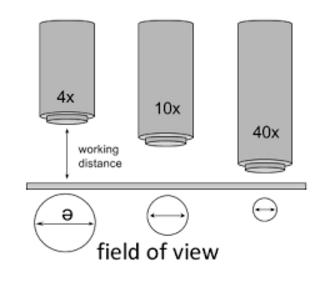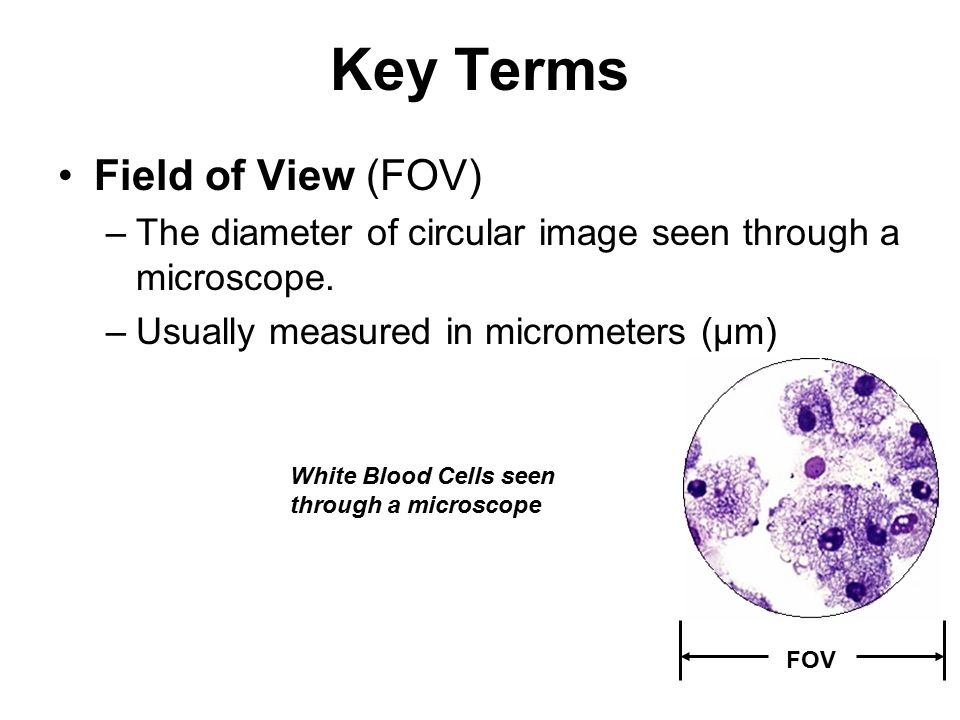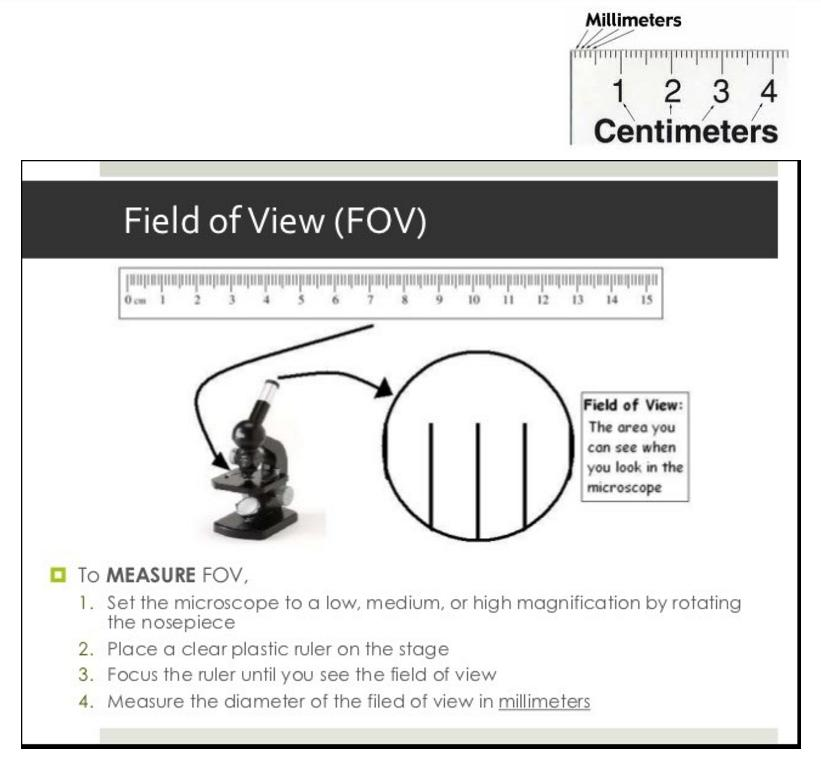IV - Microscopes - Determining Field of View
starstarstarstarstarstarstarstarstarstar
by Kathy Egbert
| 16 Questions
1
1
Review: You are given a picture of a microorganism and told it is magnified 100 times (written 100x). Which of the objective lens on your microscope was used to get this magnification?
Total magnification is calculated by multiplying the ocular lens (10x) magnification by the magnification of the objective
A 4x, scanning objective
B 10x, low power
C 40x, high power
The area (called Field of View) you can see through the microscope lens gets smaller and smaller with greater magnification.2
1
Review: Even though you have seen this question before, it is essential that you understand this principle when viewing specimens under the microscope. The greatest field of view is at what magnification?
A 4x, scanning
B 10x, low power
C 40x, high power
Here is another image showing how the field of view changes with greater magnification. Notice that the depth of the field of view also decreases greatly with an increase in magnification.Definition of Field of ViewBecause the microscope measures things that are small, we use a smaller unit of measurement to make measurements more accurate. The unit of measurement is called the micrometer. You need to know how to convert millimeters into micrometers.3
1
To increase accuracy of measuring specimens under the microscope we use the micrometer unit of measurement.
True
False
Metric System ReviewYou will need to convert millimeters (mm) into micrometers4
1
Practice: Given the measurement 3.25 mm, convert this to micrometers.
multiply 3.25 mm by 1000
A 3250 micrometers
B 325.0 micrometers
C 32.5 micrometers
Calculating Field of View
You are now ready to begin measuring and calculating the field of view on your microscope and completing your table. Every microscope can have a slightly different field of view, so it will be important that you continue using the same microscope for measuring the field of view in this worksheet.5
0
After lining up a millimeter mark on the ruler on one side of the diameter, two things can happen: 1) the millimeter marks can frame both sides; just count the number of whole millimeters (look at column 1 below) 2) the millimeter mark frames one side, but not the other (see column 2 below). Count the whole mm marks first. Now you have to estimate the amount of area that remains. Does it look like about 1/4, 1/3, 1/2 or ___? Add this fraction to the whole number. You need to have the fraction in decimal format (1/2=0.5; 1/4=0.25; 1/3=0.33; 1/2=0.5; 2/3=0.66; 3/4=0.75)
Practice Measuring Diameter6
1
Figure A measures 4 mm
True
False
7
1
Figure B measures almost exactly 5 mm
True
False
8
1
Figure C measures 1.3 mm
True
False
9
15
Table 1. Dimensions of the Field of View Complete Column 1 (a, b, c) by following the instructions in the above slides and entering measured mm; Complete Column 2 (d, e, f) convert the mm in column 1 to micrometers by multiplying by 1000 (this is the diameter); Column 3 (g, h, i) - to get the radius you divide the diameter by two, this is "r" in the equation, use 3.14 for pi and complete the multiplication for area Box X: complete this calculation by taking the low power field of view (Box b) and dividing it by 4 Box Y: convert diameter in Box X to micrometers Box Z: use same information for Column 3 above
10
1
Compare the estimated high power FOV area (Box i) with your calculated high power FOV area (Box z). Which of the following statements best represents your results
A Estimated area is much larger than the calculated area (i > z)
B Estimated area is about the same as calculated area (i = z)
C Estimated area is much smaller than the calculated area (i < z)
11
1
A Estimated and Calculated areas for the high power FOV were close to the same number, so I did a good job measuring the FOV with the ruler.
B Estimated and Calculated areas for the high power FOV were very different. I need to improve my measuring skills.
You now have the FOV for all three objective lens (4x, 10x, and 40x) on your microscope because you have recorded them in Table 1, boxes g, h, and i -- Now, let's put that information to work
12
3
Prepare a wet mount from a piece of newsprint that has some color on it. Start with the scanning, 4x objective and bring your specimen into focus. Observe how the colors are printed onto the wood fibers that make up the newspaper. Using the information you've just calculated for FOV, estimate the number of colored dots that are in one square millimeter. Choose just one of the three FOVs to do this task. Show your work:
Another tidbit of knowledge: Like FOV (Field of View), light intensity also decreases with higher magnification; observe how the light intensity changes in the schematic below. This is one of the reasons that you may have to change the amount of light coming in through the diaphragm.13
5
You will now use three different prepared slides from the front table to practice your skills. Complete a Table for each slide. Specimen #1
14
5
Repeat with a different slide. Specimen #2
15
5
Repeat with another different slide. Specimen #3
16
5
One of those rare opportunities to earn some extra credit. Repeat using a different slide - Specimen #4